# Implementation details¶

This section describes the technical details of the simulation flow for a standard model component and for the conversion of a Chandra observation. In order to take advantage of the efficient array manipulation capabilities provided by numpy, the entire implementation is vectorized, i.e. we don’t have an explicit event loop in python.

## Standard model component¶

The basic flow of the simulation for a single standard model component is coded in ixpeobssim.srcmodel.roi.xModelComponentBase.rvs_event_list(). Mathematically speaking, the simulation algorithm can be spelled out in the form of the following basic sequence:

1. Given the source spectrum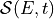and the effective area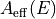, we calculate the count spectrum as a function of the energy and time: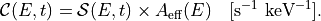In case of a source with cosmological redshift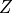or hydrogen column densitydifferent from zero, these two quantities are used to correctly re-scale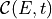: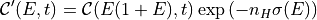2. We calculate the light curve of the model component (in counts space) by integrating over the energy: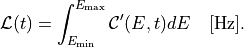3. We calculate the total number of expected events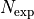by integrating the count rate over the observation time:and we extract the number of observed events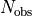according to a Poisson distribution with mean.

4. We treat the count rate as a one-dimensional probability density function in the random variable, we extract a vectorofvalues ofaccording to this pdf—and we sort the vector itself. (Here and in the following we shall use the hat to indicate vectors of lenght.)

5. We treat the array of count spectra, evaluated at the time array, as an array of one-dimensional pdf objects, from which we extract a corresponding array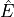of (true) energy values. (In an event-driven formulation this would be equivalent to loop over the values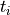of the array, calculate the corresponding count spectrum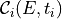and treat that as a one-dimensional pdf from which we extract a (true) energy value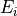, but the vectorized description is more germane to what the code is actually doing internally.)

6. We treat the energy dispersion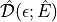as an array of one-dimensional pdf objects that we use to extract the measured energiesand the corresponding PHA values.

7. We extract suitable arrays of (true)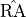,values and, similarly to what we do with the energy dispersion, we smear them with the PSF in order to get the correponding measured quantities.

8. We use the polarization degree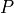and angleof the model component to calculate the visibility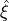and the phase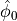of the azimuthal distribution modulation, given the modulation factor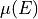of the polarimeter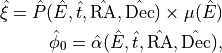and we use these values to extract the directions of emission of the photoelectron.

(For periodic sources all of the above is done in phase, rather than in time, and the latter is recovered at the very end using the source ephemeris, but other than that there is no real difference.)

For source models involving more than one component, this is done for each component separately, and the different resulting phothon lists are then merged and ordered in time at the end of the process.

## Conversion of a Chandra observation¶

In this case the starting point is a Chandra photon list, publicly available on the on-line database, which includes temporal, energetic and spatial information for each detected event. Being the Chandra angular and energetic resolution (respectively HPD1 arcsec and FWHM5% at 5.9 keV) much better than those expected for the IXPE mission), energiesand positions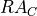,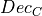measured by Chandra can be assumed as Monte Carlo truth.

From the implementation standpoint, the conversion is coded in ixpeobssim.srcmodel.roi.xChandraObservation.rvs_event_list() and can be summarized in the following steps:

1. We compute the ratiobetween the Chandra observation timeand the provided IXPE simulation time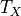: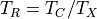2. We scale Chandra detected events by first concatenating the measured arrays (,and) as many times as the integer part of(denoted) and then appending to the end of the sequence a portion whose relative length is equal to the fractional part of(called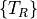). For instance, in case of the energy: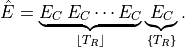3. We calculate the effective area ratio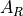between one IXPE telescope and Chandra as a function of energy and off-axis angle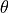:4. This ratio allows to conveniently down-sample the Chandra time-scaled photon list. In practice, this is done by throwing an array of random numbers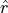between 0 and 1, and accepting as IXPE events only those that meet the condition: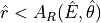5. We randomly extract the event timesuniformly between the starting and ending observation time.

6. From this point, once the arrays,,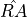and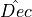of true values are selected, the simulation goes ahead as in the standard model component case. In particular, we smear these quantities with the IXPE response functions and we extract the emission anglebased on the input polarization model.

In case of definition of multiple sub-regions of the ROI, the conversion is done separately for each of them (skipping those flagged as to be removed), and the resulting photon lists are merged and ordered in time at the end of the process.